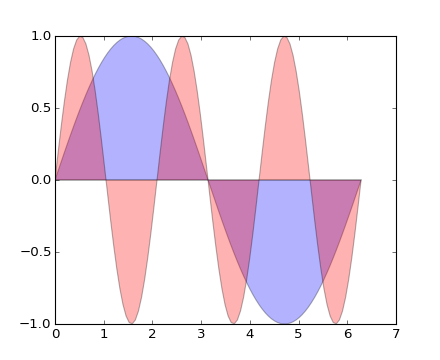### We're updating the default styles for Matplotlib 2.0

Learn what to expect in the new updates#### Previous topic

lines_bars_and_markers example code: fill_demo.py

#### Next topic

lines_bars_and_markers example code: line_demo_dash_control.py

# lines_bars_and_markers example code: fill_demo_features.py¶```"""
Demo of the fill function with a few features.

In addition to the basic fill plot, this demo shows a few optional features:

* Multiple curves with a single command.
* Setting the fill color.
* Setting the opacity (alpha value).
"""
import numpy as np
import matplotlib.pyplot as plt

x = np.linspace(0, 2 * np.pi, 100)
y1 = np.sin(x)
y2 = np.sin(3 * x)
plt.fill(x, y1, 'b', x, y2, 'r', alpha=0.3)
plt.show()
```

Keywords: python, matplotlib, pylab, example, codex (see Search examples)